Want to share your content on R-bloggers? click here if you have a blog, or here if you don't.

Here is a simulation I run with R in the same period I created the poker one.

I have just decided to call it plain vanilla since neither double down or split pairs are allowed. Rules are as basic as they can be.

The code looks like messy, I know, If I had to do it now, I guess I would do many things differently, however, the results looks fine, and the code runs fine as well, therefore it is not entirely to be thrown away I believe.

The simulation is divided in two parts. In the first one I looked for the probabilities for each possible event (that’s to say: “player wins”, “tie”, “dealer wins”). Since the code looks pretty hairy, many explanations are provided.

A small but important note: if you want to run the simulation, since it is a bit demanding computationally speaking, in order not to crash RStudio you may want to run a line a time and not the entire code all at once, or just maybe use only some functions, just be careful because since we run the simulation a big number of times and the functions are not the fastest to be run, your computer might complain a little.

Here is the code:

Let’s have a look at the results that we have got: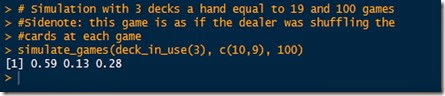By simply standing with a hand equal to 19, you can expect to win 59% of the times, and loose 28%. A tie is likely to occur 13% of the times.

Let’s now simulate 2000*100 games with a hand equal to 18 and 3 decks and then average the probabilities:In this case the odds seem to be a lot worse than before, by just subtracting 1 point to the player’s hand we raised the probability of win for the dealer from 28% to 43.4%.

Why not run the simulation for each hand from 16 to 21 and then plot the results? Warning: your computer may yell at you after this demanding step!As we could expect, as the player’s hand gets higher, the probabilities of winning increases. For some reason which I ignore, R did not print in plain the probabilities of winning for the dealer, however you can easily get them from the table above or by calculating for each row 1 – Prob of Win – Prob of a tie, since the three events should (must) sum up to one. It is interesting to note that the probability of a tie is more or less near 12-14% and that, as we expected, it is equal to 0 when the hand is equal to 16 (since the dealer must draw on 16 and therefore a tie is not possible).

Here are the plots: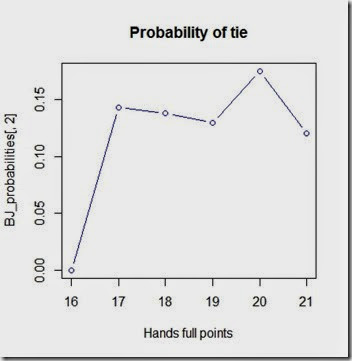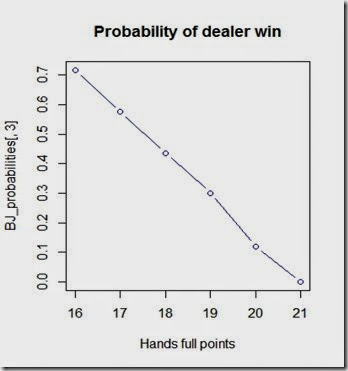This is the end of part 1 of the simulation.

And now the second part. Here we want to know what are the probabilities of not bust when we draw 1 or 2 cards given a certain hand.

Let’s have a look at the new results we have got:
This is a hand we have got by asking 2 times card from a single deck.with a starting hand equal to (10,”A”) if we were to ask 2 cards, here are the probabilities that we should face:Eventually, we can compute the probabilities of “survival” and bust for each hand from 16 to 17 and plot the results.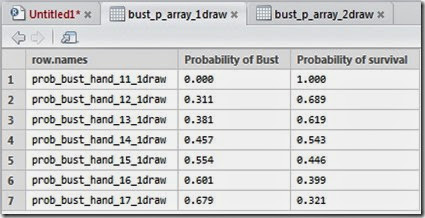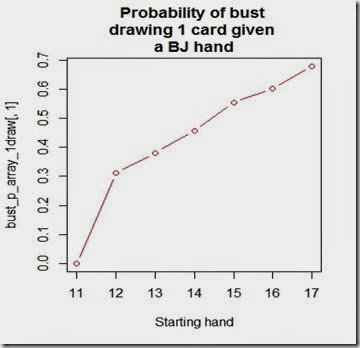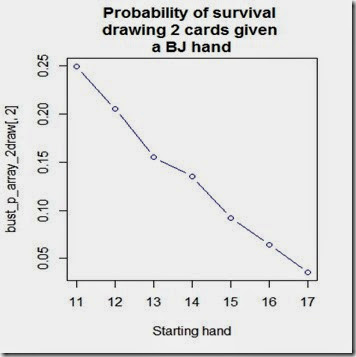The results looks pretty interesting and it is nice to see that the probability of bust if we ask for 1 card and our starting hand is 11 is 0 as we could easily predict, however, if we were to ask 2 cards, our chances of survival would suddenly drop to 25%. A big leap!

Hope this was interesting.

Disclaimer: This article is for educational purpose ONLY. Odds generated by this code are calculated by a random simulation. As such the odds will represent an approximation of the true odds. They might even be completely wrong or misleading. This code must NOT be used for anything other than educational purpose. The provider of this code does not guarantee the accuracy of the results and accepts no liability for any loss or damage that may occur as a result of the use of this code. Understanding and agreeing to the terms of this disclaimer is a condition of use of this code. By reading the article you confirm you have understood and will comply with this disclaimer.